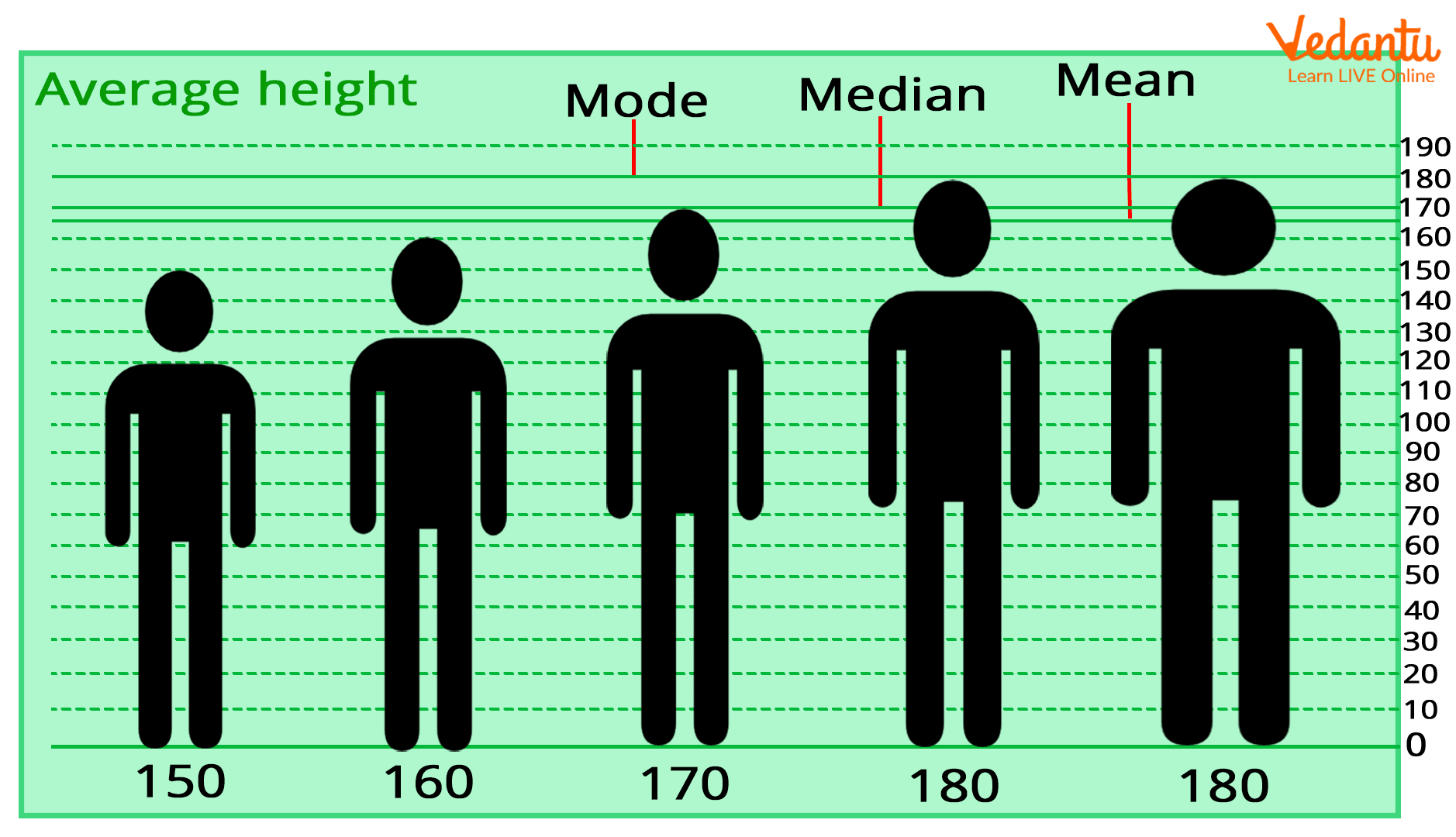Courses
Courses for Kids
Free study material
Free LIVE classes
More

# Learn Concept of Average and Types with Examples for Kids## What is an Average?

Last updated date: 23rd Mar 2023
Total views: 78k
Views today: 0.56k

Let us assume that you go to the market along with two of your friends to purchase a gift item for your science teacher. Suddenly, you find a weighing machine in the shop and each of you decides to see your weight, so your weight comes out to be 4 kg, your friend F1 has 6 kg, and another friend F2 has 5 kg. Then the average of your weight would be the sum of your weights divided by 3 (your 2 friends and you).

Is there any other way to show the average?

## Types of Averages

The three types of averages are as follows:

Mean: It is obtained by adding all the numbers and dividing the sum by the total numbers in the given set.

Median: It is the middle number from the given set of numbers.

Mode: It is the most common result.Average types

Moving forward, we will provide an explanation of all these types.

## Explanation of Average

From the above text, we noticed the following three values of weight:

• Friend F1 - 6

• F2 - 5

Now, let us sum up these values: 4 + 6 + 5 = 15

Number of people = 3

The average weight of three kids will be calculated as:

$Average=\frac{Sum\, of\, weight\, of\, three\, people}{Number\, of\, people}=\frac{15}{3}=5$

Here, you can notice the following things:

 Your weight Friend F2 weight Friend F1 weight 4 5 6

Just add 4 and 6 = 4 + 6 = 10

Now, divide it by two people = $\frac{10}{2}= 5$

Again, you get ‘5’ here, which means your friend’s weight is the average of you and your friend F1’s weight. Also, you can say that F2 is the mean weight of you and your friend.

Hence, you and your friend’s F1 weight is close to or approximately F2’s weight.

Besides this, we call the middle value ‘5’ of the given set of three numbers the median of the set.

## How to Find Averages in Simple Terms?

The average of a set of numbers is simply the sum of the observations upon the total number of values in the set. For example, suppose we want to determine the median of 4, 2, 10, 8 and 6. Now let us arrange these values:

2

4

6

8

10

Simply, find the sum of the numbers: 2 + 4 + 6 + 8 + 10 = 30 and divide by 5 to get average $\left (\frac{30}{5}\right )= 6$. Here, you can notice that 6 is in the middle and the average that we got here is also 6, which means 6 is the median of the above observation.

Now, let’s discuss about the mode. The mode is the most common result. For example, Class A students have roll numbers in the following order:

• Rohit - 10

• Sonali - 15

• Rishabh - 20

• Sohan - 25

Now, adding these numbers- 10 + 15 + 20 + 25 = 60

Now, dividing the sum by 4:

$\frac{120}{8} = 15$

Let us take another class. Class B has students’ roll numbers in the following order:

• Roma - 6

• Shreya - 9

• Nitya - 15

• Suhani - 20

• Rohan - 25

• Ruhan - 15

• Rina - 15

• Reena - 15

Summing up the observations - 6 + 9 + 15 + 20 + 25 + 15 + 15 + 15 = 120

Dividing the sum by 8, we get $\frac{120}{8} = 15$

Now, comparing the average of the roll numbers of Class A and B, we see that Class B has students with 15 marks, which is the repetitive data and in such a case when the particular data is the most frequent in a set of observations, we call it the mode of the observation. Here, 15 is the mode of the observation of Class B.

So, this was all about the average, median and mode. Here, we did two examples by summing up the observations and then dividing them. Also, we compared the two cases to understand the concept of mode. This is how we can understand the average types with real-life examples.

## FAQs on Learn Concept of Average and Types with Examples for Kids

1. How are averages used in everyday life?

We use the term ‘average’ in everyday life to express an amount that is typical for a group of people or things. For example, you may notice people watching cricket matches and mostly it is said that on an average five people watch the match 3 hours per day.

2. How do you explain average to kids?

In Maths, we know that the average value in a set of numbers is the middle value or the mean of the observations to the left and the right of the middle number. It is calculated by dividing the total of the set of numbers by the given number of values.